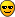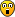Support

If you have a problem or need to report a bug please email : support@dsprobotics.com

There are 3 sections to this support area:

HELP & INFORMATION: tutorials and example files for learning or finding pre-made modules for your projects

USER FORUMS: meet with other users and exchange ideas, you can also get help and assistance here

DSP related issues, mathematics, processing and techniques

Howdee,

the subject of using bitmasks in FS e.g. to implement logical structures comes up every now and then so I thought perhaps a tutorial might be helpful to have. I plan to do this in a number of installments so this is only the first part of a series. Please feel free to interrupt me and post questions.

It is always a good idea to consult the User Guide first, so let me start with that:ConditionalStatements.png (41.4 KiB) Viewed 24839 times

Let's elaborate a bit. There is the & operator, a "bitwise and" for the arguments left and right of it. In the example given, one argument is a boolean expression (x >= 1) and the other is a float number 1.0. Both have a 32 bit representation in FS per SSE channel. The boolean expression is either true or false:

Code: Select all
`true  = 11111111111111111111111111111111false = 00000000000000000000000000000000`

Float numbers are represented as bit patterns according to the IEEE 754 standard, which for the example happens to be

Code: Select all
`1.0 = 00111111100000000000000000000000`

"Bitwise and" means that each of the 32 binary digits is evaluated to 1 if both those digits are 1, and is zero otherwise. At the end of the day, all you need to remember is the following:

Code: Select all
`number & true  = numbernumber & false = 0`

By the way, the order of the arguments does not matter, so bool & number is the same as number & bool.martinvicanek

Posts: 1080
Joined: Sat Jun 22, 2013 8:28 pm

### Re: Bitmasking in DSP Code

This is going to be interesting and useful!Thanks

SpoggSpogg

Posts: 2554
Joined: Thu Nov 20, 2014 4:24 pm
Location: Birmingham, England

### Re: Bitmasking in DSP Code

Time for some schoolin'. Thanks MartinRJHollins

Posts: 1411
Joined: Thu Mar 08, 2012 7:58 pm

### 2. Examples

So let's look at some working examples.
Our first example will be a (naive, non bandlimited) sawtooth oscillator with frequency f and the waveform going from -1 to +1.

C code would look something like this:
Code: Select all
`float f = 0.1;float saw = 0;saw = saw + f;if (saw > 1.0) saw = saw - 2.0;`

This translates to the following FS code:
Code: Select all
`float f = 0.1;float saw = 0;saw = saw + f;saw = saw - 2&(saw > 1);`
Easy, isn't it?

Next consider a simple index ramp. A C code would look something like this:
Code: Select all
`integer i = 0;integer n = 10;i = i + 1;if (i >= n) i = 0;`

The FS code box offers only floating point declarations, so it would read
Code: Select all
`float i = 0;float n = 10;i = i + 1;i = i&(i < n);`
Note that we have negated the comparison in FS in this case.

So how do we implement an if-then-else structure? For instance
Code: Select all
`if (x > y) then z = a;else z = b;`

In FS you could write
Code: Select all
`z = a&(x > y) + b&(x <= y);`

This expression is correct, however it involves two comparisons. A more efficient implementation is
Code: Select all
`z = b + (a - b)&(x > y);`
which requires only one comparison. To see why this gives the same result assume (x > y) is true, then z = b + (a - b) = a. If, on the other hand, (x > y) is false then z = b + 0 = b.

Note: You could also write
Code: Select all
`z = a + (b - a)&(x <= y);`
Last edited by martinvicanek on Thu Sep 21, 2017 7:03 am, edited 1 time in total.martinvicanek

Posts: 1080
Joined: Sat Jun 22, 2013 8:28 pm

### Re: Bitmasking in DSP Code

First of all: Thank you for taking the time to start at the very beginning!

Unfortunately, I already am overchallenged with the last two code examples. While it is easy to comprehend when it is already there, I just don't know how I would get from the two-condintional version to the one-conditional version on my own. I'm missing the steps involved to get from
Code: Select all
`z = a&(x > y) + b&(x <= y);`

to
Code: Select all
`z = b + (a - b)&(x > y);`

Because that doesn't come naturally to me. But exactly those are the things that make code more efficient.
If you feel like gifting: https://paypal.me/tulamide
tulamide

Posts: 2196
Joined: Sat Jun 21, 2014 2:48 pm
Location: Germany

### Re: Bitmasking in DSP Code

Look at it this way: In the instruction
Code: Select all
`z = b + (a - b)&(x > y);`
the first term b is the "default" value whereas the second term (a - b) represents a correction in the case that the condition (x > y) is true. In prose you could say:

If nothing else then set z equal to b.
However, in the event that (x > y) replace it by a.
Do so by adding a and subtracting b.

Of course you can reverse the roles of a and b if you prefer a to be the "default":
Code: Select all
`z = a + (b - a)&(x <= y);`

Did it help?martinvicanek

Posts: 1080
Joined: Sat Jun 22, 2013 8:28 pm

### Re: Bitmasking in DSP Code

However to make things even more complicated, the first example with two comparisons is actually more efficient in assembly I believe. In assembly it could look like this:

Code: Select all
`z = a&(x > y) + b&(x <= y);movaps xmm0,x;       //we are moving 'x' to register xmm0cmpps xmm0,y,2;      //we compare if x is <= y then we get the bitmask, either true or falsemovaps xmm1,xmm0; //we store this bitmask to another registerandps xmm0,b;         //b value will be active if condition is met (and)andnps xmm1,a;       //a is active if the inverse condition is met (and not)andps xmm0,xmm1;   //then we choose the correct value`

You can see we used only one comparison and also andnps so we didnt use any addition or subtraction which use more cpu than 'and' or 'and not'

Posts: 501
Joined: Sun Jul 11, 2010 7:21 am

### Re: Bitmasking in DSP Code

Adam, you are miles ahead of me!Yes, if you do it in assembly you have more options, for instance you can use andnps. But watch out, the negation applies to the first argument! (You have that right in your code). The other thing is that it may not work with older FS versions, dunno when they added that opcode.

In (older) textbooks you read that bit operations are faster than floating point operations (and that floating point adds are faster than multiplies). I am not sure if this is still valid with modern CPUs, so I tested your ASM implemetation against my z = b + (a - b)&(x > y); ASM implementation - and they were tie!

Anyway, let's not frighten the audience with assembly nitpicking, there is still some DSP code material ahead.martinvicanek

Posts: 1080
Joined: Sat Jun 22, 2013 8:28 pm

### Re: Bitmasking in DSP Code

and we [I] are easily frightened !RJHollins

Posts: 1411
Joined: Thu Mar 08, 2012 7:58 pm

### 3. More Booleans and the "or" Operator

The arguments of of the & operator need not be a number and a boolean, respectvely. For instance you might want to combine two booleans. An example is the following zero crossing detector:
Code: Select all
`streamin in;    // current samplefloat in1;      // previous samplefloat zx;       // boolean, true if zero crossing just happenedzx  = (in > 0)&(in1 <= 0);in1 = in;`
Note that FS allows you to store booleans, even though the declared datatype is float.

In addition to the & operator, FS also has an "or" operator denoted by |. This can be useful to combine boolean expressions. Suppose you want to start off a timer triggered by an impulse at the input. you could use the above expression for zero crossing, however it is more efficient to work with the negated expression (in <= 0)|(in1 > 0):
Code: Select all
`streamin in;    // input trigger pulsefloat in1;      // previous input valuefloat time;     // elapsed time (in samples) since last triggertime = time + 1;time = time&((in <= 0)|(in1 > 0));   // reset timer on triggerin1  = in;`martinvicanek

Posts: 1080
Joined: Sat Jun 22, 2013 8:28 pm

Next